# Sallen-Key Low-pass Filter Design Tool

This page is a web application that design a Sallen-Key low-pass filter. Use this utility to simulate the Transfer Function for filters at a given frequency, damping ratio ζ, Q or values of R and C. The response of the filter is displayed on graphs, showing Bode diagram, Nyquist diagram, Impulse response and Step response.

## Calculate the transfer function for Sallen-Key low-pass filter with R and C values

 Vin(s)→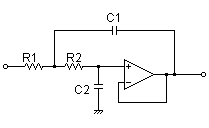→Vout(s)
Transfer function: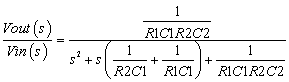R1=Ω C1=F R2=Ω C2=F
p:pico, n:nano, u:micro, k:kilo, M:mega

### Frequency analysis

Bode diagram
Phase  Group delay
Nyquist diagram
Pole, zero
Phase margin
Oscillation analysis

Upper and lower frequency limits:
f1= - f2=[Hz] (frequency limits are optional)

### Transient analysis

Step response
Impulse response
Overshoot
Final value of the step response

Simulation time:
0 - [sec] (optional)

## Calculate the R and C values for the Sallen-Key filter at a given frequency and Q factor

 Vin(s)→→Vout(s)
Cut-off frequency: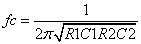Transfer function:fc=Hz

### Q factor | Damping ratio ζ

Quality factor Q =
Damping ratio ζ =
 C1 = F C2 = F
C1, C2 is optional. But when setting these capacitances, C1 and C2 of both are needed to give following the equation
(C2/C1) ≤ ζ 2
(C1/C2) ≥ 4Q2

Select Capacitor Sequence:
Select Resistor Sequence:

### Frequency analysis

Bode diagram
Phase  Group delay
Nyquist diagram
Pole, zero
Phase margin
Oscillation analysis

Upper and lower frequency limits:
f1= - f2=[Hz] (frequency limits are optional)

### Transient analysis

Step response
Impulse response
Overshoot
Final value of the step response

Simulation time:
0 - [sec] (optional)

## Calculate the transfer function for Sallen-Key low-pass filter with R and C values

 Vin(s)→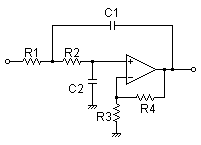→Vout(s)
Transfer function:
Transfer function
 R1=Ω C1=F R2=Ω C2=F R3=Ω R4=Ω
p:pico, n:nano, u:micro, k:kilo, M:mega

### Frequency analysis

Bode diagram
Phase  Group delay
Nyquist diagram
Pole, zero
Phase margin
Oscillation analysis

Upper and lower frequency limits:
f1= - f2=[Hz] (frequency limits are optional)

### Transient analysis

Step response
Impulse response
Overshoot
Final value of the step response

Simulation time:
0 - [sec] (optional)

## Calculate the R and C values for the Sallen-Key filter at a given frequency and Q factor

 Vin(s)→→Vout(s)
Cut-off frequency:Transfer function: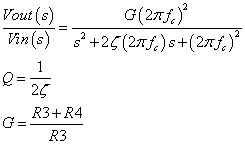fc=Hz
G= at f=0Hz (G>1)

### Q factor | Damping ratio ζ

Quality factor Q =
Damping ratio ζ =
 C1 = F C2 = F
C1, C2 is optional. But when setting these capacitances, C1 and C2 of both are needed to give following the equation
G-1 < C2/C1 ≤ ζ 2+G-1

Select Capacitor Sequence:
Select Resistor Sequence:

### Frequency analysis

Bode diagram
Phase  Group delay
Nyquist diagram
Pole, zero
Phase margin
Oscillation analysis

Upper and lower frequency limits:
f1= - f2=[Hz] (frequency limits are optional)

### Transient analysis

Step response
Impulse response
Overshoot
Final value of the step response

Simulation time:
0 - [sec] (optional)